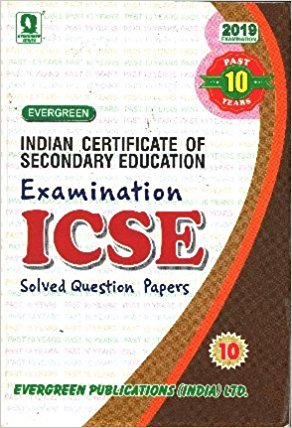# Java X ICSE Solutions

Q 1. Write a program to print following pattern using BLUE J IDE.

1234321
123*321
12***21
1*****1

Ans :

Following program is tried and tested in Blue J 3.08 version.

class jaiii
{
public static void main(String args[])

{
int i,j,l;

for(i=4;i>=1;i–)
{

for(j=1;j<=i;j++)
System.out.print(j);
for(int m=i;m<4;m++) //increment o *
System.out.print(“*”);

for(int n=3;n>i;n–) //decrement of *
System.out.print(“*”);
for(l=i;l>=1;l–)
{ if(l==4)
continue;
System.out.print(l);
}
System.out.println();
}

Q 2.  WAP to print following pattern in java

A
A B
A B C
A B C D
A B C D E

Ans : Given program below is test on Blue J 3.08 version .

public class sid6

{

public static void main(String args[])

{

char ch;

for(int a=1;a<=5;a++)

{

ch=’A’;

for(int c=4;c>=a-1;c–)

{

System.out.print(” “);

}

for(int b=1;b<=a;b++)

{

System.out.print(ch+” “);

ch++;

}

System.out.println();

}

}

}

Now its the time to buy the 10 year question paper for ICSE examination.  Almost all the portions

are over for 10th graders. I suggest now every student of ICSE board to practice questions from the old sample papers and do the time bound tests. You will definitely improve your efficiency in coming days. Any questions or

doubts you can reach to me.